## Slide physics

Posted by Kpoluk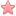12 Apr 2020 in 12:23
Before we dig into slide theme let's examine too related techniques – WallRun and WallSlide.

### WallRun

In the article about strafe physics we found that if you run with W than periodic pressing of strafe button for 15-30 frames leads to oscillation of speed in range of 249-262 units/s. Trajectory won't be linear, but on the average you move faster this way, that's why it's called FastRun.

But this is not the only way to run faster on the ground without ducks and bhops. When you run along the wall with pressed W and start to turn towards it you can notice that your speed is more then 250 units/s. This technique is called WallRun. Under the hood we have the same effect as we had during lj prestrafe. The only difference is that each frame velocity `Vnew`, which was received as addition of current velocity `V` and increment `dV`, is projected onto the plane of wall, thanks to PM_ClipVelocity, that we faced in the article about EdgeBug and JumpBug:Here the wall is located on the right and vector `dV` has direction of our view cause we pressed only W. Unlike running without wall we don't have to turn our mouse all the time trying to keep a certain angle between line of view and velocity, cause velocity is always directed along the wall. If we recall 3D graph of speed gain depending on angle `u` we find ourslef on the edge of pit starting from 250 units/s. Now let's increase angle `u` between `dV` and velocity `V` i.e. turn more towards the wall. Then on 3D graph we crawl along the edge of the pit, moving to the area of higher speed and larger angle `u`. The wall doesn't allows us to fall into the pit, and we safely reach 277 units/s at angle `u` about 27°:Unfortunately futher magnification of `u` forces us to descend into the area of lower speed, but reaching the maximum prestrafe with so little effort is really astonishing.

Of course we can have the same result not with W only. For instance, simultaneously pressed W and D give the same direction of `dV` if we look 45° to the left than just with W.

### WallSlide

This technique is also known as kkz jump by the nick of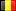koukouz who actively used it in his world records. Nevertheless it was used before as well. The difference from WallRun is that movement takes place in the air, so we should press D instead of W (we still suppose the wall is located to the right). At that velocity is still projected to the plane of the wall every frame, hence we don't have to do fast mouse movements as we did with regular air strafes. It's enough just to keep angle `u` in the range that gives speed gain. In the article about LongJump physics we learned that this range is from `u > arccos(30 / V)` to `u < arccos(-12.5 / V)` with maximum gain at `u = arccos(5 / V)`. In case of WallSlide we maximize not the velocity `Vnew`, but its projection to the wall plane `Vx = Vnew * cos(x)`, where `x` is the angle between `Vnew` and `V`:Growth of `Vx` also starts at `u > arccos(30 / V)`, but stops already at `u = 90°`, which is clear from the picture. At `u` from `arccos(5 / V)` to 90° growth of `Vx` is still present, but goes down. Therefore optimal `u` that maximizes `Vx` is somewhere between `arccos(30 / V)` and `arccos(5 / V)`. We can find it with help of cosine theorem as we did before, but this time we write it relative to the angle `x`:

`dV^2 = V^2 + Vnew^2 - 2 * V * Vnew * cos(x)`

Substitute here results from the article about LongJump physics: `dV = 30 - V * cos(u)` and `Vnew^2 = V^2 * sin(u)^2 + 900`:

```900 - 60 * V * cos(u) + V^2 * cos(u)^2 = V^2 + V^2 * sin(u)^2 + 900 - 2 * V * Vnew * cos(x) Vx = Vnew * cos(x) = -V * cos(u)^2 + 30 * cos(u) + 1```

Replacement `z = cos(u)` leads us to the funtion `Vx(z) = -V * z^2 + 30 * z + 1`, which has maximum at `z = 15 / V`, hence optimal angle `u = arccos(15 / V)`.

As we did for air strafes let's find some specific values of `u` for a set of speeds `V`:Actually since we don't use strafes switching we could make all reasoning not for the angle `u` between `dV` and `V`, but for the angle between line of view and plane of the wall, which is `90 - u`. For example, at the speed of `V = 250` units/s maximum gain happens when we are turned `90 - 86.56 = 3.44°` from the wall. If we turn away more then `90 - 83.11 = 6.89°` our velocity won't change. Angles are so small that layman may say that player just looks along the wall, but now we know what happens in fact.

WallSlide is not just an alternative to the LongJump, but may be the only way to perform a jump. Representative situation is when you need to jump in a narrow long corridor that has no space for a winding trajectory of lj. As an example let's examine 2 jumps by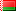throttle from his demo on prochallenge2_mix (you can download the demo here). On the last climb stage throttle has 19:14 on timer and he's jumping 230 block, sandwitched between two walls. Width of model is 32 units, while the distance between walls is 36 units, so trying lj is very problematic there. That's why throttle uses right wall firstly for WallRun with W and D, and then for WallSlide with D.GIF 2.23MB

Note that he turned mouse to the right before the jump so in the air he's already in the range of `u` which gives a speed gain for WallSlide. Of course he paid with speed losses for it at the end of WallRun. During the flight throttle didn't take risks and kept mouse static, so angle `u` was remaining the same.

Literally 10 seconds later throttle does another jump which is way more harder. It's located above 230 block, so aperture is the same. However one cannot do WallRun here, so he had to jump around the corner and then cling to the right wall:GIF 1.80MB

As we can see during the flight throttle by inertia touched the left wall first, which gave him a gray sector on the left chart (within these frames velocity vector didn't change either magnitude or direction). Next not yet touching the right wall he turned mouse to the left, so another gray sector here. And finally after touch he has stable speed gain with the help of WallSlide.

Another good example is Stand-Up CountJump in a narrow corridor on qcg_to_chichin. Its mapper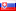Qicg recorded for us this jump (you can download demo here).GIF 3.68MB

The aperture here is just 34 units. Qicg also uses right wall, accelerates with W and D, then performs double duck with squat, releases duck right before landing, spends 6 frames on the ground, jumps and implements Wallslide with held D. So many FOG (frames on the ground) were made not by chance, it allowed to significantly diminish jumpoff, i.e. to jump closer to the edge of small block. At that with regular Stand-Up CountJump Qicg would lost a lot of speed on the ground, but here he preferred to turn mouse towards the right wall yet after double duck, so during 6 frames on the ground he actually continued to do WallRun, gaining the speed. With more FOG jumpoff would be even less, although then it would be difficult to call it Stand-Up CountJump.

And one more demonstration of active WallSlide use is a demo byfykseN on sn_beachcliff (you can download demo here ). There are a lot of closely spaced blocks along the rocks, where WallSlide is useful both for single jumps and for bhop.

### Slide

In the article about EdgeBug and JumpBug we learned from function PM_CategorizePosition that slope becomes slide when tilt angle `a` is more then `arccos(0.7) = 45.573°`. Game engine processes slide as air movement, but unlike it was with WallSlide the gravity is very important element here. Funtions PM_AddCorrectGravity and PM_FixupGravityVelocity from the article about bhop physics showed that every frame the gravity subtracts from vertical speed 8 units/s. We will represent it as addition of vector `dVz` which is 8 units long and directed straight down. Resulting velocity will be found as a sum of current velocity `V`, vertical increment from gravity `dVz` and horizontal increment `dV` from function PM_AirAccelerate. The remarkable thing is that magnitude of `dV` depends only on horizontal part of current velocity, so `dVz` (which is added in code before `dV`) doesn't affect it. After addition the resulting vector is projected onto the plane of slide by function PM_ClipVelocity. Already here we can make an important conclusion that for slide it doesn't matter you look up or down, changing of velocity is entirely defined only by turning in horizontal plane, i.e. right-left.

#### Vertical movement

Let's begin from a simple case – come to the slide (not too steep) and start ascending with held W (look straight towards the slide to avoid sideways movement):At first speed is low, hence increment `dV` is at a peak of 25. If projection of `dV` onto the plane of slide is more then projection of `dVz`, then resulting speed increases. In fact it immediately gives us a condition under which the rise is possible:

```dVz * sin(a) < dV * cos(a) 8 * sin(a) < 25 * cos(a) a < arctg(25 / 8) = 72.255°```

If `a >= 72.255°` we deal with so called Steep Slide, where sliding down is inevitable. On the other hand on a regular slide our speed keeps growing and soon `dV` is calculated already as `30 - V * cos(a)` and starts to decline. As soon as `dV` is equal to `dVz * sin(a)`, changing of speed stops, and we will continue to crawl up the slide steadily. We could use another way – run and jump to the slide holding W, then at first there won't be any speed increase, but soon it will reaching the value of `dVz * sin(a)`, and finally we will have the same constant speed while sliding up.

But can we slide up in duck? In the article about CountJump physics we found out that in duck variables `pmove->cmd.forwardmove` and `pmove->cmd.sidemove` are multiplied by 0.333, hence in PM_AirAccelerate `accelspeed = 10 * 0.01 * (250 * 0.333) * 1 = 8.325`, i.e. maximum increment `dV = 8.325`. It means that slide in duck possible at `a < arctg(8.325 / 8) = 46.141°`. And since slide starts at `a > 45.573°`, mapper should get into a range of less then 1°, that's why such slides are quite rare to meet. Anyhow, required angle may be not even on the slide itself, but at the junction of slides.

Here are representative sizes of slide blocks on some maps:slide_gs_longjumps (`a = 48.814°`)slide_svn_steepslides `(a = 72.662°`)kz-endo_slide_svn_duckslides (`a = 48.366°`)slide_svn_longslides (`a = 45.881°`)

Be aware that on b2j_slide_longjumps, which is a logical follow-up of slide_gs_longjumps, block sizes are a bit different – the height of start platform is 257, not 256 units, which suggests that tilt angle is `a = 48.925°`. Similar situation happens on qcg_longslides, which can be considered by block sizes as continuation of slide_svn_longslides – horizontal dimension of slide here is 129, not 128 units, hence tilt angle is `a = 45.659°`.

Also notice that slide on kz-endo_slide_svn_duckslides is placed 64 units above the ground. But we already know that maximum height we can jump on is 63 units! Strange right? We will solve this puzzle in the next article, while now let's move on.

#### Horizontal movement

Another special case is when we move along the slide holding `D` and having strictly horizontal velocity in the plane of slide:Velocity `V` and increment `dV` lay in horizontal plane, while increment `dVz` is directed vertically down. We'll try to understand how real is to maintain this state without sliding up or down. In other words after addition of `V`, `dV` and `dVz` and projecting the result onto the slide plane we need to receive strictly horizontal velocity. Sounds difficult but if we split `dV` into two parts (along velocity `V` and perpendicular to it), then things clear up. Component along `V` will give an augment to horizontal velocity, while perpendicular component should balance `dVz`:

`dVz * sin(a) = dV * sin(u) * cos(a)`

In the range of `u` from `acrcos(5 / V)` to `90°` (i.e. `cos(u)` from `0` to `5 / V`) increment `dV = 25`, and this condition looks like

`8 * tg(a) = 25 * sin(u)`

After calculating `u` at various `а` we find out that condition `V < 5 / cos(u)` leads to limiting the speed down to 5-6 units/s. However since speed `V` constantly grows at `u < 90°`, we cannot maintain this state.

Thus `u` should be in the range from `acrcos(30 / V)` to `acrcos(5 / V)`, where increment `dV = 30 - V * cos(u)`. Substitute this increment into the balance condition:

`8 * tg(a) = (30 - V * cos(u)) * sin(u)`

We won't try to solve this equation for `u`, we'll just mention that along with growth of `V` angle `u` should be enlarged (means we should turn towards the slide little by little).

#### Turning towards slide

If during the horizontal movement along slide we start to turn mouse towards slide (angle of turning away from horizontal direction of slide will be denoted as `y`), then velocity will turn in the same direction, staying in slide plane:Magnitude of `dV` still depends on angle `u`, which is still counted from horizontal component of velocity (violet vector on the picture), directed inside the slide block. It means that if we want to keep `dV` nonzero, we should continue to turn towards the slide in next frames, which is very similar to logic of air strafes.

In order to understand what's going on with velocity `V` we decompose it into two components in slide plane (dark red vectors on the picture). Also we decompose Increment `dV` in horizontal plane and thus we can see that one part of `dV` will slow down horizontal movement along the slide, while the other part will try to balance gravitional increment `dVz`. Unless angle `y` is too big we are slowing down in horizontal direction of slide and accelerate up the slide.

As an example examine the demo by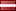BuTaMuH on b2j_slide_longjumps with 241 slide block (you can download it here). Follow the change of horizontal speed and angle `u` from the moment of jump to the landing:GIF 3.84MB

You can clearly see how red vector of horizontal velocity bounces at the moment of slide touch – the whole velocity is projected to the slide plane. After the touch BuTaMuH continues to turn mouse towards the slide increasing horizontal speed and slowing slide down at the same time. Thereby at the lowest point speed reaches 749.3 units/s. During slide up horizontal speed is steadily decreasing while vertical speed is growing, which can be seen on this graph:Here we have gain of vertical speed starting from the lowest point and up to the last frame on the slide. The angle of turing towards the slide was changing from `y = 0°` to `y = 50°`. Taking the maximum increment `dV = 25` at `y = 0°` we find that gain of vertical speed is `dV * cos(y) * cos(a) * sin(a) = 25 * cos(0) * cos(48.925°) * sin(48.925°) = 7.84` units/s, while at `y = 50°` we have 4.53 units/s. In case of BuTaMuH one could already see from animation that angle `u` firstly deviated from `arccos(5 / V)` downwards, leading to decrease of `dV` and a little pit at the beginning of the graph. Futher angle `u` became more than `arccos(5 / V)`, so BuTaMuH had maximum gain of vertical speed, but at the same time he started to lose speed into horizontal direction of slide. Before leaving the slide BuTaMuH turned mouse away from the slide in advance, so increment `dV` plummeted, and gravitation outweighed. At the last frame on slide horizontal speed was 83.3 untis/s, while vertical one was 387 units/s.

Note: values of speed are stored in demo file with an error of 0.125 units/s, thereby graph calculated as differences of such values has error of 0.25 units/s. In this regard graph hit the level of 8.0 while the maximum possible gain is 7.84 units/s.

#### Turning away from slide

In this article we've aleady get acquainted with steep slide, and now it's time to explore the last block on slide_svn_steepslides_h performed during the run by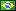.script (you can download the demo here):GIF 3.43MB

After air flight and touching the slide .script carries out familiar procedure – he turns towards the slide losing speed and ascending. But after passing the top point a curious thing happens – as he continues to slide he gradually turns away from the slide. It is a necessary thing cause after passing the top point he begins to slide down more and more (as we remember gravity always wins on steep slide). Increasing vertical velocity is projected onto the plane of the slide and becomes a horizontal one, hence resulting horizontal velocity turnes away from the slide. And since .script wants to maintain speed gain, he's turing his mouse to the left trying to keep angle `u` in the required range.

#### Conclusion

When we tried to assess human possibilities for lj we've already had hard times, but in case of slide it's close to impossible. The reason is that we face simultaneous changes in horizontal and vertical velocities, and even when considering a specific jump as we just did, the result will greatly depend on the initial and final heights on the slide, as well as on how the player will manage the gained speed after the slide. In random case both sizes and tilt angle of slide vary, so the final goal may be to fly as high as possible, or gain high horizontal speed, or something average like we saw on b2j_slide_longjumps. That is why you can meet maps in kreedz which are almost entirely dedicated to slide. Despite the fact that we consider the slide as just one of the techniques, it may act as an independent direction, while its boundaries of the possible remain quite blurred.

You can recall how in the article about LongJump physics we discussed the existance of special servers with setting sv_airaccelerate changed from 10 to 100. In case of slide this at first glance small difference led to the formation of entire communities dedicated to surf (this is how slide with sv_airaccelerate 100 was called). This is due to the fact that firstly maximum increment `dV` becomes equal to 30 instead of 25, which affects gaining of both horizontal and vertical velocities. The longer we stay on surf the more sv_airaccelerate difference plays a role, allowing to reach huge speeds. Secondly the range of angle `u` that gives gain of horizontal speed is wider, which lets surfers to be more maneuverable in flight.

That's it for now, but we'll back to the theme of slide later.